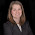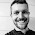## Friday, February 24, 2012

### Simulating Genetic Drift

In population genetics, the neutral theory of evolution "claims that most of DNA sequence difference between alleles within a population or between species are due to neutral mutations" [Population Genetics: A Concise Guide by John Gillespie, 2nd edition].  Under this model, genetic mutations insert genetic variation into populations and are countered by the process of genetic drift which eliminates genetic variation from populations.  A very simple model of genetic drift can be simulated using the Wright-Fisher model.  Below is simulation of genetic drift using the Wright-Fisher model written in R.

Consider N = 20 diploid individuals with two segregating sites A1 and A2 with probability 0.20 and 0.80, respectively.  Let X be the number of A1 alleles.

## Simulate Genetic Drift (using Wright-Fisher model)
library(ggplot2)
library(reshape)

# Set up parameters
N = 20 # number of diploid individuals
N.chrom = 2*N # number of chromosomes
p = .2; q = 1-p
N.gen = 100 # number of generations
N.sim = 5 # number of simulations

# Simulation
X = array(0, dim=c(N.gen,N.sim))
X[1,] = rep(N.chrom*p,N.sim) # initialize number of A1 alleles in first generation
for(j in 1:N.sim){
for(i in 2:N.gen){
X[i,j] = rbinom(1,N.chrom,prob=X[i-1,j]/N.chrom)
}
}
X = data.frame(X/N.chrom)

# Reshape data and plot the 5 simulations
sim_data <- melt(X)
ggplot(sim_data, aes(x = rep(c(1:100), N.sim), y = value, colour = variable)) + geom_line() + opts(title = "Simulations of Genetic Drift") + xlab("Generation") + ylab("Allele Frequency") + ylim(0,1) + labs(colour = "Simulations")

We plot the frequency of the A1 allele for 100 generations for 5 different simulations given the initial allele frequency for A1 is 0.20.  The plot show that 3 out of the 5 simulations resulted in the A1 allele being lost from the population and 1 out of the 5 result in the A2 allele being lost from the population.  There is also one simulation which resulted in the A1 allele still in the population after 100 generations. This is an example of how genetic drift removed variation from populations.

Note: there are many other tools (e.g. Hudson, 2002, simuPOP) out there (with available software) that will simulate more complicated versions of genetic drift.

1.I note that N.chrom is constant. Then is an allele an observed characteristic, rather than the presence or absence of a chromosome? So is it correct to conclude that though low probability allele's may disappear over generations, the genetic materials remain such that they may serve as raw materials for a mutation?

1.Yes. N.chrom being fixed is an assumption of the Wright-Fisher model. An allele represents a nucleotide (A,T,C,G) at a particular position in the genome. Typically you talk about a pair of alleles at a segregating site (i.e. at a particular position in the genome some people have the A1 allele and other have the A2 allele with some probability p(A1), p(A2) ). The idea of genetic drift is that whenever there is genetic variation introduced such as at a segregating site, then genetic drift will remove that variation over time. As you would suspect, neutral evolution is not what we observe in real life because other factors come into play such as selection. Lots of models have been created to try and incorporate it.

2.Yes. the low and high probabilities translate to the next generation and then from that generation to next, which will eventually cause one to be lost and the other to be fixed respectively.

3.You may also show on a separate code the fluctuations due to sampling effects. I copied this code from my molecular evolution class:
> pop1 <- c(rep("A",500), rep("G",500)); pop2 <- c(rep("A",100), rep("G",900))
> hg = function(x) {
+ cts = table(x); total=sum(cts);
+ if (length(cts)==1) {return(0) }
+ else { freq1=cts/total; freq2=cts/total;
+ return(1-(freq1^2+freq2^2)) }
+ }
#evaluate 100 samples from population 1
> pop1<-sample(pop1); s <- sample(pop1, 100); counts<-table(s); heterozygosity <- hg(s)
> heterozygosity
You can do the same for population 2 and then you can change the sample size.

2.I do have problems with plotting the data i created with your simulation.
I am kinda new to R so i couldn't work out any solution by myself so far.
The error i am getting:
Aesthetics must either be length one, or the same length as the dataProblems:1:100
I would appreciate any help regarding this matter.

Sincerly,

Flo.

1.Flo,

Thank you for your question. There was an error in the code: In the line that starts with ggplot2 'x = 1:100' should be replaced with 'x = rep(c(1:100), N.sim)'. It has since been corrected in the code.

Hope this helps
Stephanie

2.Hello Stephanie,

thank you for your help regarding this matter. When i copy the code it works perfectly fine but i still have issues with my version. I am currently trying to work out, why problems still are there^^
But thanks to you i have a version i can work with.

With best regards,

Flo.

3.I've changed mine to 'x = rep(c(1:N.gen)' so that I can alter N.gen with different simulations and then it updates the plot dimensions automatically.

3.So for X[i,j] = rbinom(1,N.chrom,prob=X[i-1,j]/N.chrom). Why is there a 1 in the rbinom command? Just wondering what that space means? Thanks

4.This comment has been removed by the author.

5.bpalm,

The 1 in 'rbinom(1,N.chrom,prob=X[i-1,j]/N.chrom)' means to randomly sample 1 observation from the binomial distribution with parameters n, p where n = N.chrom and p = X[i-1,j]/N.chrom.

Hope this helps.
- Stephanie